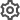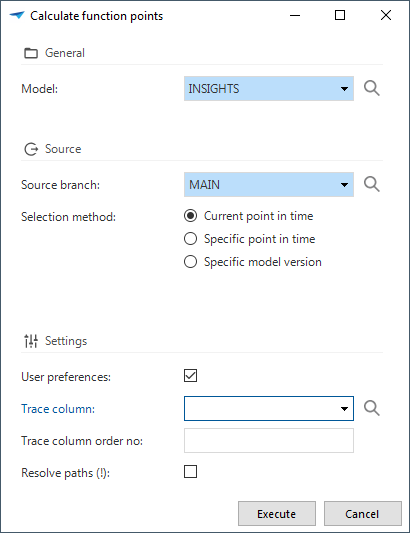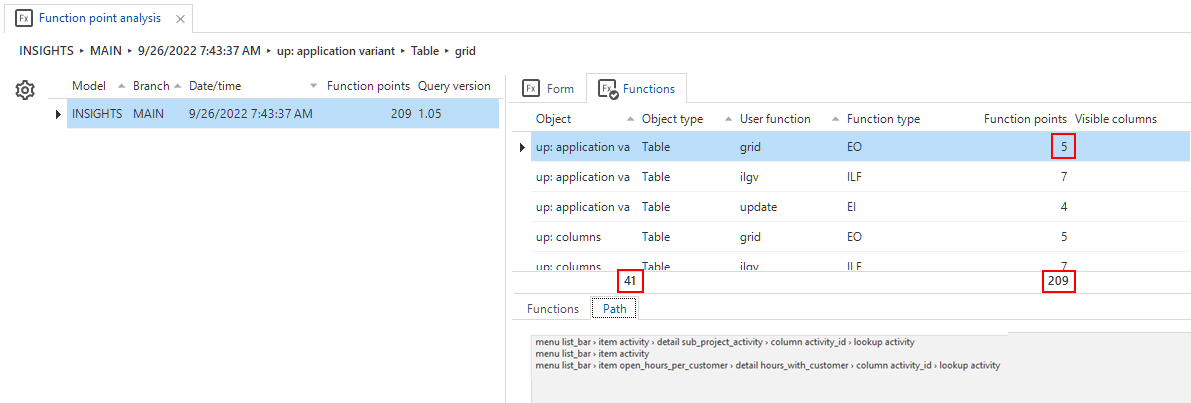Version: 2023

# Function point analysis

## Introduction to function point analysis​

In the Thinkwise platform, it is possible to automatically calculate the number of function points for your application. A function point is a unit of measurement that expresses the amount of business functionality an information system provides to a user. Function points are used to compute a functional size measurement of software. (Source: wikipedia)

The automated function point analysis of the Thinkwise Platform has been developed in collaboration with and verified by QSM, the leading authority on Quantitative Software Management. It satisfies the Nesma counting practices at a global level.

By analyzing the model, the function point analysis determines exactly which data and transactional functions are offered to a user by the user interface. Points are assigned to each of these functions to calculate the total number of function points.

The analysis:

• identifies and counts internal logical data collections, taking into account cardinalities and optionalities
• identifies and counts FPA tables
• identifies and counts User Transactions
• Input functions
• Output functions
• Inquiry functions
• assumes primary ILFs and looks for the associated functionality that is implemented in the specific model
• eliminates double counts.

## Calculate function points​

menu Analysis > Function point analysis

1. Execute the Calculate function pointstask.

2. Select a Model and a Branch.

3. Select for which situation you want to calculate the function points:

• The current point in time
• A specific model version
• A custom point in time.
4. Select if User preferences are available in your end-product. This adds extra function points.

5. If trace columns are used in a separate tab or form component, select the name of one of these columns in the field Trace column. Based on that column, it is determined whether a table contains trace columns. If so, an extra External Inquiry (EQ) function will be added for this table.

6. The Trace column order is the order number of the first trace column. Trace columns are ignored when determining if a table is a code table.

7. To calculate the navigation path from the menu to every object (table, task, report), select Resolve paths (!). Note that this may take a long time. The navigation paths provide information to validate the assigned points.Calculate the function points

In tab Functions, you can view the number of objects that have been analyzed, the function points that have been assigned to each object, and the total number of function points. In tab Path, the calculated paths are available.Example function point analysis